Ch 3. Particle Energy Methods Multimedia Engineering Dynamics Work &Energy Conservative Forces Power & Efficiency
 Chapter - Particle - 1. General Motion 2. Force & Accel. 3. Energy 4. Momentum - Rigid Body - 5. General Motion 6. Force & Accel. 7. Energy 8. Momentum 9. 3-D Motion 10. Vibrations Appendix Basic Math Units Basic Equations Sections Search eBooks Dynamics Fluids Math Mechanics Statics Thermodynamics Author(s): Kurt Gramoll ©Kurt GramollDYNAMICS - THEORY

Work and Energy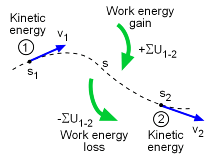Energy Balance
T1 + ΣU1-2 = T2

An alternate method to solve many dynamic problems is the energy method. This method is particularly helpful if the problem involves finding position or velocity when two different positions along its motion path are known.

Basically, energy methods analyze all energy added or deleted as an object moves between two points. The difference in energy between the two points must be accounted for with an increase or decrease in kinetic energy (i.e. velocity). In equation form, this is stated as,

 T1 + ΣU1-2 = T2

where
T1 = kinetic energy at position 1 = ½mv12
T2 = kinetic energy at position 2 = ½mv22
ΣU1-2 = total change in energy (i.e. work)
between positions 1 and 2

Energy methods are a convenient method to solve for velocity or position. However, energy methods do not work for acceleration.

Notice, energy terms are scalars which do not have direction. The energy equation is not a vector equation. This means that only one unknown can be determined.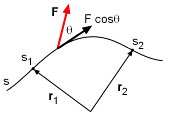Work Energy over Path "s"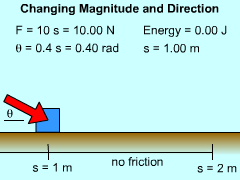Example with Changing Force
and Direction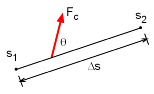Work Energy over Path "s"

General Work

The most common energy generated in a dynamics problem is a force, F, acting through a distance (or more acurately, a change in position, r). This written in equation form as

ΔU = F•dr

The dot product is needed since only the force in the direction of the motion will produce work.

To calculate the total work, integrate the work over the motion path. The total work done from position 1 to position 2 isNotice that F and the angle θ can change over the path.

When F and θ are constant, the work equation simplifies to

U1-2 = Fc cosθ (s2 - s1) = Fc cosθ Δs

Positive work means the force is acting in the direction of the motion (i.e. increasing its velocity). Negative work is when the force is acting opposite the motion (i.e. reducing its velocity).

Gravity Work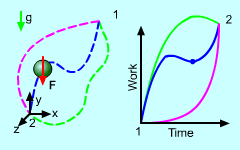Work on Object Due to Gravity is
Independent of Path

Gravitational force, mg, is a constant force that always points downward. The work energy equation simplifies to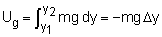Δy is the change in vertical direction (up is positive)

Since gravitational force is perpendicular to x and z directions no work is generated in horizontal directions.

Spring Force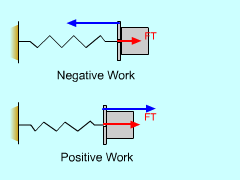Spring Work

The basic force on a spring is proportional to the change of the spring length,

F = ks

Since the spring force is a variable force, it needs to be integrate over the distance, s, giving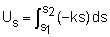Us = ½k(s22 - s12)

Kinetic Energy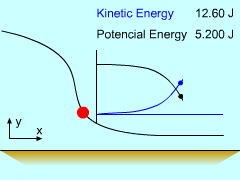Potential and Kinetic Energy

The motion of an object is also stored energy and is called kinetic energy. This can be illustrated by applying a simple force, F, on an object over a distance, s, and finding its final velocity. In other words, the change in work (F Δs) will produce a change in kinetic energy, or simply

ΔUkinetic = F Δs

dUkinetic = Fds

Using the relationships, "F = ma" and, "a ds = v dv", gives,

dUkinetic = m(v dv/ds) ds = mv dv

Integrating both sides gives,

Ukinetic = ½mv2

Thus the kinetic energy, T, for any object in motion is

 T = ½mv2

Practice Homework and Test problems now available in the 'Eng Dynamics' mobile app
Includes over 400 problems with complete detailed solutions.
Available now at the Google Play Store and Apple App Store.